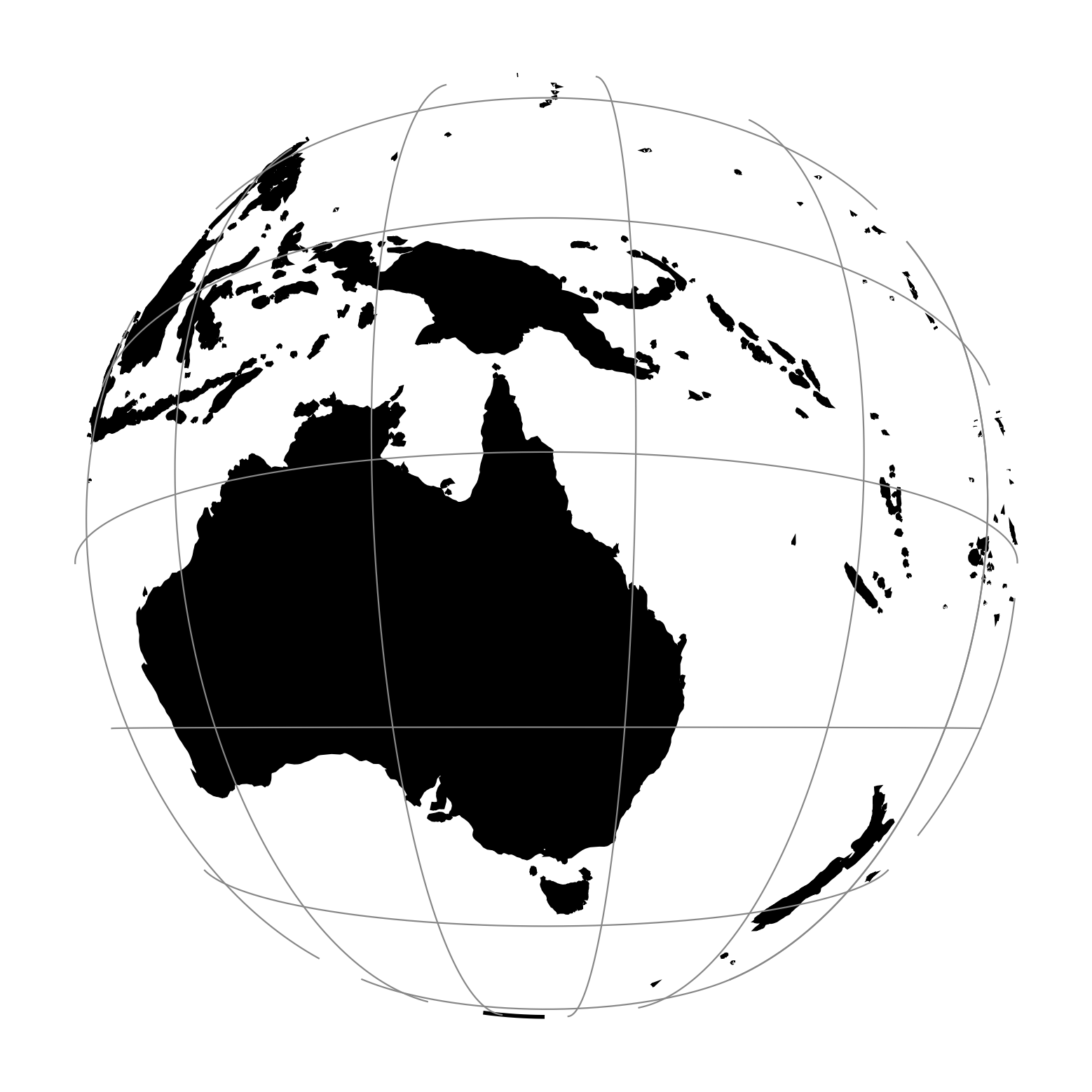# Near-sided perspective¶

The near-sided perspective projection simulates a view from a height $$h$$ similar to how a satellite in orbit would see it.

 Classification Azimuthal. Neither conformal nor equal area. Available forms Forward and inverse spherical projection Defined area Global, although for one hemisphere at a time. Alias nsper Domain 2D Input type Geodetic coordinates Output type Projected coordinatesproj-string: +proj=nsper +h=3000000 +lat_0=-20 +lon_0=145

## Parameters¶

### Required¶

+h=<value>

Height of the view point above the Earth and must be in the same units as the radius of the sphere or semimajor axis of the ellipsoid.

### Optional¶

+lon_0=<value>

Longitude of projection center.

Defaults to 0.0.

+R=<value>

Radius of the sphere given in meters. If used in conjunction with +ellps +R takes precedence.

+x_0=<value>

False easting.

Defaults to 0.0.

+y_0=<value>

False northing.

Defaults to 0.0.